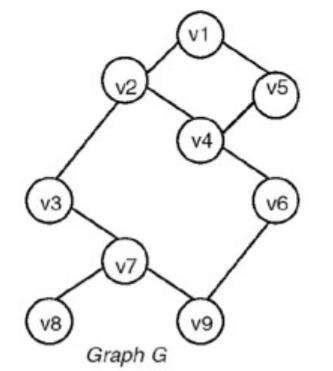When a graph is traversed by visiting all the adjacent nodes/vertices of a node/vertex first, the traversal is called breadth-first traversal. For example, for a graph in which the breadth-first traversal starts at vertex v1, visits to the nodes take place in the order v1 v2 v5 v3 v4 v7 v6 v8 v9.Breadth First Traversal for a graph is similar to Breadth First Traversal of a tree. The only catch here is, unlike trees, graphs may contain cycles, so we may come to the same node again. To avoid processing a node more than once, we use a boolean visited array. For simplicity, it is assumed that all vertices are reachable from the starting vertex.

Following are C++ , Python and Java implementations of simple Breadth First Traversal from a given source.

The C++ implementation uses adjacency list representation of graphs. STL‘s list container is used to store lists of adjacent nodes and queue of nodes needed for BFS traversal.

## C++

```// Program to print BFS traversal from a given
// source vertex. BFS(int s) traverses vertices
// reachable from s.
#include<iostream>
#include <list>

using namespace std;

// This class represents a directed graph using
class Graph
{
int V;    // No. of vertices

// Pointer to an array containing adjacency
// lists
public:
Graph(int V);  // Constructor

// function to add an edge to graph

// prints BFS traversal from a given source s
void BFS(int s);
};

Graph::Graph(int V)
{
this->V = V;
}

{
}

void Graph::BFS(int s)
{
// Mark all the vertices as not visited
bool *visited = new bool[V];
for(int i = 0; i < V; i++)
visited[i] = false;

// Create a queue for BFS
list<int> queue;

// Mark the current node as visited and enqueue it
visited[s] = true;
queue.push_back(s);

// 'i' will be used to get all adjacent
// vertices of a vertex
list<int>::iterator i;

while(!queue.empty())
{
// Dequeue a vertex from queue and print it
s = queue.front();
cout << s << " ";
queue.pop_front();

// Get all adjacent vertices of the dequeued
// vertex s. If a adjacent has not been visited,
// then mark it visited and enqueue it
{
if (!visited[*i])
{
visited[*i] = true;
queue.push_back(*i);
}
}
}
}

// Driver program to test methods of graph class
int main()
{
// Create a graph given in the above diagram
Graph g(4);

cout << "Following is Breadth First Traversal "
<< "(starting from vertex 2) \n";
g.BFS(2);

return 0;
}
```

## Java

```// Java program to print BFS traversal from a given source vertex.
// BFS(int s) traverses vertices reachable from s.
import java.io.*;
import java.util.*;

// This class represents a directed graph using adjacency list
// representation
class Graph
{
private int V;   // No. of vertices

// Constructor
Graph(int v)
{
V = v;
for (int i=0; i<v; ++i)
}

// Function to add an edge into the graph
{
}

// prints BFS traversal from a given source s
void BFS(int s)
{
// Mark all the vertices as not visited(By default
// set as false)
boolean visited[] = new boolean[V];

// Create a queue for BFS

// Mark the current node as visited and enqueue it
visited[s]=true;

while (queue.size() != 0)
{
// Dequeue a vertex from queue and print it
s = queue.poll();
System.out.print(s+" ");

// Get all adjacent vertices of the dequeued vertex s
// If a adjacent has not been visited, then mark it
// visited and enqueue it
while (i.hasNext())
{
int n = i.next();
if (!visited[n])
{
visited[n] = true;
}
}
}
}

// Driver method to
public static void main(String args[])
{
Graph g = new Graph(4);

System.out.println("Following is Breadth First Traversal "+
"(starting from vertex 2)");

g.BFS(2);
}
}
// This code is contributed by Aakash Hasija
```

## Python

```# Program to print BFS traversal from a given source
# vertex. BFS(int s) traverses vertices reachable
# from s.
from collections import defaultdict

# This class represents a directed graph using adjacency
# list representation
class Graph:

# Constructor
def __init__(self):

# default dictionary to store graph
self.graph = defaultdict(list)

# function to add an edge to graph
self.graph[u].append(v)

# Function to print a BFS of graph
def BFS(self, s):

# Mark all the vertices as not visited
visited = [False]*(len(self.graph))

# Create a queue for BFS
queue = []

# Mark the source node as visited and enqueue it
queue.append(s)
visited[s] = True

while queue:

# Dequeue a vertex from queue and print it
s = queue.pop(0)
print s,

# Get all adjacent vertices of the dequeued
# vertex s. If a adjacent has not been visited,
# then mark it visited and enqueue it
for i in self.graph[s]:
if visited[i] == False:
queue.append(i)
visited[i] = True

# Driver code
# Create a graph given in the above diagram
g = Graph()

print "Following is Breadth First Traversal (starting from vertex 2)"
g.BFS(2)Output:
```
```Following is Breadth First Traversal (starting from vertex 2)
2 0 3 1
```

Note that the above code traverses only the vertices reachable from a given source vertex. All the vertices may not be reachable from a given vertex (example Disconnected graph). To print all the vertices, we can modify the BFS function to do traversal starting from all nodes one by one.

Time Complexity: O(V+E) where V is number of vertices in the graph and E is number of edges in the graph.

### Relevant exercises

POST A NEW COMMENT

• Input (stdin)

Output (stdout)

Input (stdin)

Expected Output

Compiler Message

Input (stdin)

`2    3`

`5`
`5`
`5`